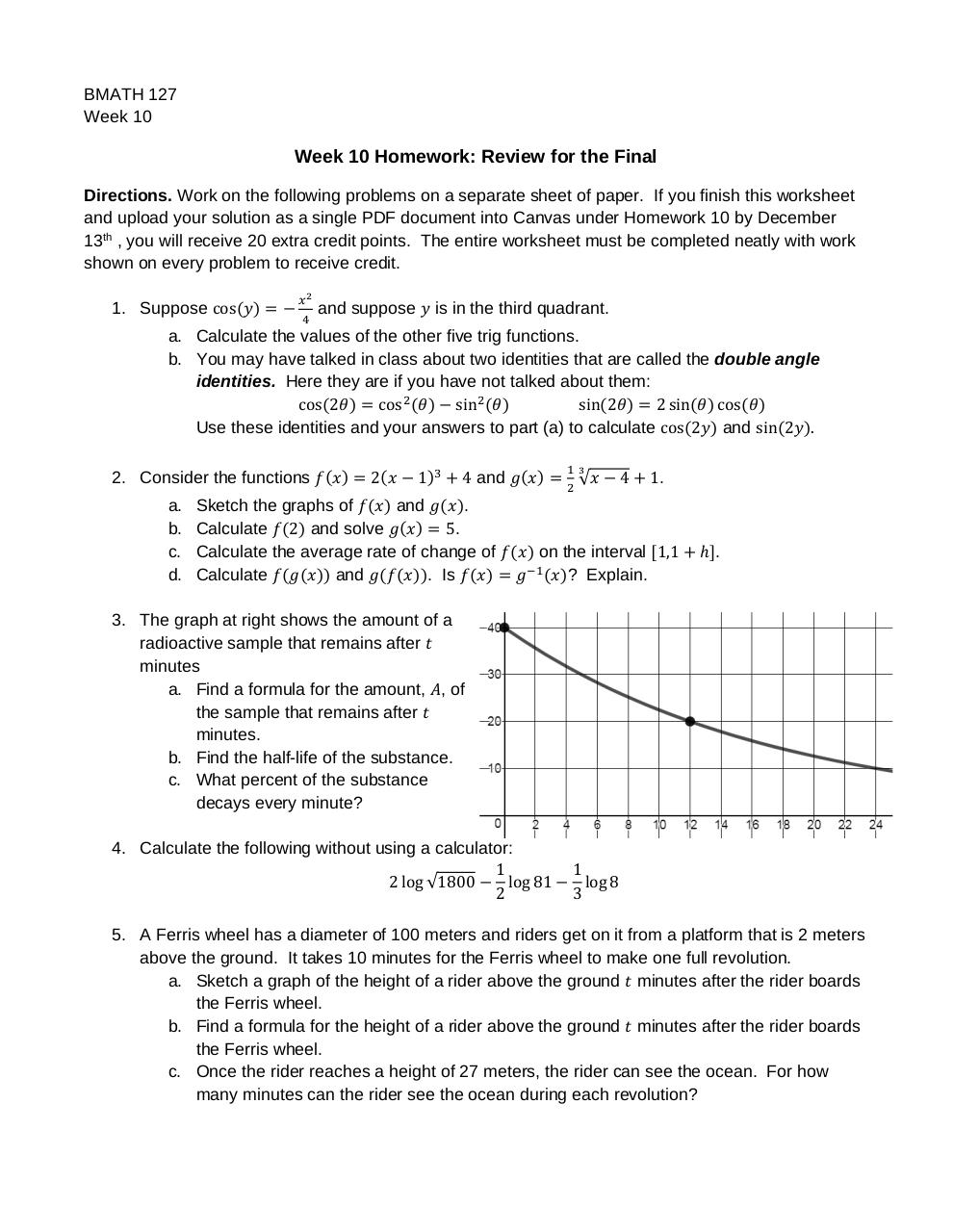# Week10homework .pdf

### File information

Original filename: Week10homework.pdf
Title: Microsoft Word - Week10homework.docx
Author: EGismervig

This PDF 1.5 document has been generated by PScript5.dll Version 5.2.2 / Acrobat Distiller 9.5.5 (Windows), and has been sent on pdf-archive.com on 08/12/2017 at 03:10, from IP address 69.91.x.x. The current document download page has been viewed 167 times.
File size: 46 KB (1 page).
Privacy: public file

Week10homework.pdf (PDF, 46 KB)

### Document preview

BMATH 127
Week 10

Week 10 Homework: Review for the Final
Directions. Work on the following problems on a separate sheet of paper. If you finish this worksheet
and upload your solution as a single PDF document into Canvas under Homework 10 by December
13th , you will receive 20 extra credit points. The entire worksheet must be completed neatly with work
shown on every problem to receive credit.
1. Suppose cos

and suppose

a. Calculate the values of the other five trig functions.
b. You may have talked in class about two identities that are called the double angle
identities. Here they are if you have not talked about them:
sin
cos 2
cos
sin 2
2 sin
cos
Use these identities and your answers to part (a) to calculate cos 2 and sin 2 .
2. Consider the functions
a.
b.
c.
d.

2

1

4 and

Sketch the graphs of
and
.
Calculate 2 and solve
5.
Calculate the average rate of change of
Calculate
and
. Is

4

1.

on the interval 1,1
? Explain.

.

3. The graph at right shows the amount of a
minutes
a. Find a formula for the amount, , of
the sample that remains after
minutes.
b. Find the half-life of the substance.
c. What percent of the substance
decays every minute?
4. Calculate the following without using a calculator:
1
log 81
2 log √1800
2

1
log 8
3

5. A Ferris wheel has a diameter of 100 meters and riders get on it from a platform that is 2 meters
above the ground. It takes 10 minutes for the Ferris wheel to make one full revolution.
a. Sketch a graph of the height of a rider above the ground minutes after the rider boards
the Ferris wheel.
b. Find a formula for the height of a rider above the ground minutes after the rider boards
the Ferris wheel.
c. Once the rider reaches a height of 27 meters, the rider can see the ocean. For how
many minutes can the rider see the ocean during each revolution?#### HTML Code

Copy the following HTML code to share your document on a Website or Blog

#### QR Code### Related keywords